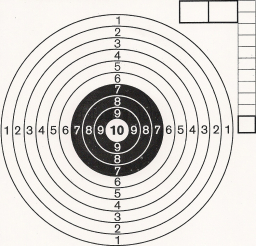# Probability 68574

The target is divided into three zones. The probability of a shooter hitting the first band is 0.18, the second band 0.2, and the third band 0.44. What is the probability that
a) hits the target,
b) miss the target?

p1 =  0.82
p2 =  0.18

### Step-by-step explanation:Did you find an error or inaccuracy? Feel free to write us. Thank you!

Tips for related online calculators
Would you like to compute the count of combinations?